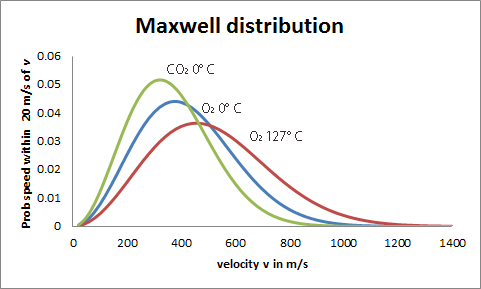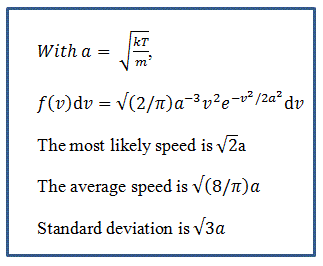The Maxwell Distribution Maxwell Legacy Concepts
In a nutshell
The Maxwell distribution is the distribution of molecular velocities in a gas at a given temperature. It underpins the general behaviour of gases. Maxwell derived this from a few simple assumptions, announcing the result at a British Association for the Advancement of Science meeting in Aberdeen, then his hometown. This and related results were published in a pioneering paper of 1860 full of insight that is seen as the beginning of statistical physics. In the 1870s Ludwig Boltzmann derived the same distribution from a more detailed discussion of the collision processes of molecules in a gas, which is why the distribution is often referred to as the Maxwell-Boltzmann distribution.Maxwell distribution of velocities in a gas. The y axis shows the probability f(v)dv of a molecule having a speed in a range of 20 m/s around a velocity on the x axis.
Technical detail

If T is the temperature of a gas (in degrees Kelvin) and m the mass of a single molecule then the only other quantity one needs to know is the fundamental constant k, known as Boltzmann's constant. The formula on the right shows how to work out the probability distribution of the molecular velocities. If there is a mixture of gases, such as is in the atmosphere, then each species has its own distribution. The Maxwell distribution of the velocity of gas molecules is an example of a statistical Χ2 distribution. The total velocity is dervied from its independant components in 3 directions at right angles. The velocity components are normally (i.e. Gaussian) distributed.

The Maxwell distribution is famous for having an extended 'tail' at high velocities, stretching out the distribution to the right in the graph. This means that there are more molecules with velocities well above the average than one might expect with, say, a Gaussian distribution. The loss of gases in the atmosphere of a planet or moon is directly dependent on the tail of the Maxwell distribution since the escape velocity is well above the average velocity in most cases. For example, Mars has lost almost all its nitrogen and any oxygen and water vapour it had but has retained some of its more masive CO2 molecules in spite of having an escape velocity of 5 km/s. (5 km/s is about 15 times the average velocity of a nitrogen molecule at the average temperature on Mars). The Earth having more than twice the escape velocity has retained most of these gases but lost its hydrogen and most of its helium.

JSR 2016